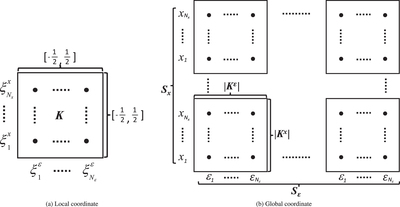Image Details

Choose export citation format:A DG-IMEX Method for Two-moment Neutrino Transport: Nonlinear Solvers for Neutrino–Matter Coupling

• Authors: A DG-IMEX Method for Two-moment Neutrino Transport: Nonlinear Solvers for Neutrino–Matter Coupling

2021 The Astrophysical Journal Supplement Series 253 52.

• Provider: AAS Journals

Caption: Figure 2.

Example of nodal DG elements in a computational domain in ﹩{\mathbb{R}}\times {{\mathbb{R}}}^{+}﹩. Figure 2(a) shows the interpolation points ﹩\{{\xi }_{j}^{i}\}{}_{j=1}^{{N}_{{\boldsymbol{x}}}}﹩ and ﹩\{{\xi }_{j}^{\varepsilon }\}{}_{j=1}^{{N}_{\varepsilon }}﹩ in a nodal DG element K in the local coordinate. Figure 2(b) shows the union ﹩{ \mathcal K }﹩ of all elements K and the space and energy nodes—the values of the interpolation points in the global coordinate. Figure 2(b) also illustrates the collection of space nodes Sx and the collection energy nodes Sε, which are defined in Equations (40) and (41).